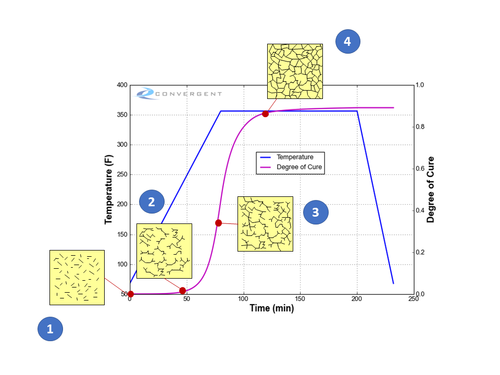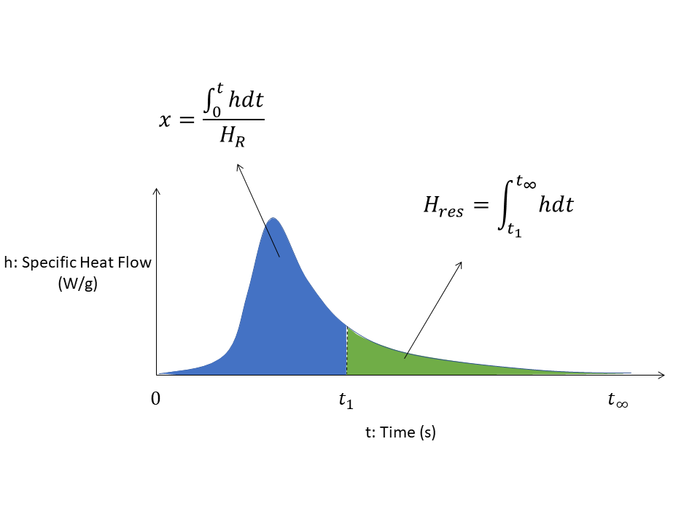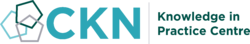The CKN Knowledge in Practice Centre is in the early stages of content creation and currently focuses on the theme of thermal management.
We appreciate any feedback or content suggestions/requests using the links below

# Degree of cure - A104

Degree of cure
Foundational knowledge articleDocument Type Article
Document Identifier 104
Themes
Relevant Class

Material

Tags
Prerequisites

## Introduction

For thermoset polymer resins (e.g. epoxies, polyesters), the degree of cure (DOC), $$x$$, is a numerical representation of how far the chemical curing reaction (crosslinking process) has advanced. It is considered a processing state variable used to describe the current polymer material state based on its process cure history.

## Scope

This page defines degree of cure and explains its significance in composites processing. Measurement and modelling methods are also briefly discussed.

## Significance

Knowledge of the degree of cure is significant for ensuring that the intended and maximum mechanical properties of the polymer matrix is achieved. The higher the degree of cure, the higher the mechanical properties for the thermoset matrix. Conversely, under curing can lead to under-strength adhesion between fibre layers, or skins and cores, and potential geometric distortions upon part removal from the tool.

In the context of composite processing, the degree of cure is one of the key state variables as it can describe the physical state of the thermoset as it transitions from a flowing resin into a solid matrix material. The degree of cure value can provide insight as to whether the polymer is still a flowing resin, has become a gelled semi-solid, or become a physical solid with some insight into the potential mechanical strength of that solid.

## Prerequisites

Recommended documents to review before, or in parallel with this document:

## Definition

For thermoset polymer resins (e.g. epoxies, polyesters), the degree of cure (DOC), $$x$$, is a numerical representation of how far the chemical curing reaction (crosslinking process) has advanced. It is considered a processing state variable used to describe the current polymer material state based on its process cure history.

### Symbol Notation

In the literature, degree of cure (DOC) is generally represented by one of the following symbols:

Symbol notation for degree of cure (DOC)
KPC preferred $$x$$
Other reported forms $$\alpha$$

### Units

Degree of cure (DOC) is a unit-less index, and is represented as either a numerical fraction or percentage (%) value.

Range Notes:
Fraction 0 - 1 where, 1 = fully cured with full crosslink network formation complete
Percentage 0% - 100% where, 100% = fully cured with full crosslink network formation complete

### Degree of Cure development during the curing process

The degree of cure (DOC) is defined as a number between 0 and 1 (or 0% and 100%), where the latter is a fully cured resin. As the curing reaction and crosslink network formation progresses, the representative DOC value likewise increases. The figure below illustrates the progression of the DOC during a typical epoxy curing cycle as the epoxy crosslinking reaction progresses. The final DOC is directly tied to the thermal cure history experienced by the resin.

As an increasing number of chemical crosslinks are formed, the DOC value increases towards 1. The DOC does not have to reach 100% (or a value of 1) to for the thermoset to be solid and in a usable state. In practice, a thermoset resin such as epoxy is considered fully cured at a DOC around 0.9 or higher. However, the higher the DOC, the higher the mechanical properties for the thermoset.

In prepreg materials, the initial as-received DOC of the thermoset matrix is not 0, instead the resin is received in a partially cured state with a low DOC and the curing reaction is continued later after shape forming.Example of the degree of cure development (crosslink network formation) during a typical thermal cure cycle.

Progression of the degree of cure (DOC) during the curing process, as indicated in the figure:

1. (DOC 0 or close to 0) Initially, the resin is comprised of short monomers prior to polymerization, and flows like a liquid.
2. With heating and time, the thermoset reaction begins with polymerization of the monomers together to form longer polymer chains and crosslinking between the polymer chains starts to occur.
3. (DOC around 0.4-0.6) The crosslink network forms to the extent that the resin’s ability to flow is restricted. At about a DOC 0.5-0.6, the resin reaches a gelled state (gelation) where resin flow stops and the resin behaves like a semi-solid gel material.
4. The resin has transformed into a solid material state. The increase in the degree of cure slows as it approaches a maximum where the majority of the potential crosslinks that can form have done so. Subsequent curing beyond this point will see a continual rise in DOC and a rise in mechanical properties until plateauing – at this point, the thermoset matrix is considered fully cured.

## Measurement

### Differential Scanning Calorimetry (DSC)

The curing reaction for thermoset polymers is exothermic in nature and generates heat. This allows the degree of cure (DOC) to be measured through differential scanning calorimetry (DSC) characterization of resin samples and is modelled as follows$x=\frac{\int_{t0}^{t1} hdt}{H_R}$

Where,

$$x=$$ Degree of cure

$$H_R=$$ Heat of reaction - total [J/g]

$$h=$$ Specific heat flow [W/g]

Visually, from a DSC characterization of the specific heat flow curve:Determining degree of cure from a DSC heat flow curve. The degree of cure of the thermoset polymer is defined as the total heat released up to that point in its cure history with respect to the total heat of reaction for full curing.

On the DSC curve, the DOC at time $$t_1$$ is the proportion of heat measured up to that point in time, compared to the full DSC run of the sample to complete cure.

Alternatively, to determine the DOC from an existing sample, a small piece of material can be measured through the DSC. Here, the residual heat is measured (the remaining unreacted portion in the above DSC curve), and is compared to a full DSC run of an initial unreacted sample - the baseline for the full amount of potential heat release of the resin system. The DOC is determined by subtracting the amount of residual heat of reaction that is obtained (unreacted portion) against the total heat of reaction (total amount of reaction possible with full curing to completion).

Here, the degree of cure is calculated as$x=1-\frac{H_{res}}{H_R}=1-\frac{\int_{t1}^{t\infty} hdt}{\int_{0}^{t\infty} hdt}=\frac{\int_{t0}^{t1} hdt}{\int_{0}^{t\infty} hdt}=\frac{\int_{t0}^{t1} hdt}{H_R}$

Where,

$$x=$$ Degree of cure

$$H_R=$$ Heat of reaction - total [J/g]

$$H_{res}=$$ Heat of reaction - residual [J/g]

$$h=$$ Specific heat flow [W/g]

#### Characterizing degree of cure (DOC) by differential scanning calorimetry (DSC)

Please see the following document on how to measure the degree of cure (DOC) as visually illustrated above, by differential scanning calorimetry (DSC) and other available means:

### Glass Transition Temperature (Tg)

The glass transition temperature (Tg) of a thermoset polymer is directly dependent on the state of its degree of cure (DOC).

A thermoset’s Tg is a reflection of its crosslink density, where the more extensive its crosslink network is formed – a higher crosslink density – the higher the observed Tg is for the thermoset polymer. As the crosslink network builds during the curing process, the thermoset polymer will correspondingly see a rise in its observed Tg. The thermoset’s maximum and final Tg is obtained when its crosslink network has formed to completion and cannot develop further.

Using this relationship, the DOC can be determined through measurement of its glass transition temperature (Tg) using the DiBenedetto equation$T_g=T_{g0}+\frac{\lambda x(T_{g\infty}-T_{g0})}{1-(1-\lambda)x}$

Where,

$$x=$$ Degree of cure

$$T_g=$$ Glass transition temperature

$$T_{g0}=$$ Glass transition temperature at 0% cure

$$\lambda=$$ Experimentally fit parameter

$$T_{g\infty}=$$ Glass transition at 100% cure

The glass transition temperature (Tg) can be measured using a variety of methods. Common laboratory methods include differential scanning calorimetry (DSC), and dynamic mechanical analysis (DMA). Note that these methods do not necessarily give the same value.

Laboratory equipment How glass transition temperature (Tg) is determined
Differential scanning calorimetry (DSC) Measure changes in heat flow
Dynamic mechanical analysis (DMA) Measure changes in viscoelastic moduli## Welcome

Welcome to the CKN Knowledge in Practice Centre (KPC). The KPC is a resource for learning and applying scientific knowledge to the practice of composites manufacturing. As you navigate around the KPC, refer back to the information on this right-hand pane as a resource for understanding the intricacies of composites processing and why the KPC is laid out in the way that it is. The following video explains the KPC approach:

## Understanding Composites Processing

The Knowledge in Practice Centre (KPC) is centered around a structured method of thinking about composite material manufacturing. From the top down, the heirarchy consists of:

The way that the material, shape, tooling & consumables and equipment (abbreviated as MSTE) interact with each other during a process step is critical to the outcome of the manufacturing step, and ultimately critical to the quality of the finished part. The interactions between MSTE during a process step can be numerous and complex, but the Knowledge in Practice Centre aims to make you aware of these interactions, understand how one parameter affects another, and understand how to analyze the problem using a systems based approach. Using this approach, the factory can then be developed with a complete understanding and control of all interactions.

## Interrelationship of Function, Shape, Material & Process

Design for manufacturing is critical to ensuring the producibility of a part. Trouble arises when it is considered too late or not at all in the design process. Conversely, process design (controlling the interactions between shape, material, tooling & consumables and equipment to achieve a desired outcome) must always consider the shape and material of the part. Ashby has developed and popularized the approach linking design (function) to the choice of material and shape, which influence the process selected and vice versa, as shown below:

Within the Knowledge in Practice Centre the same methodology is applied but the process is more fully defined by also explicitly calling out the equipment and tooling & consumables. Note that in common usage, a process which consists of many steps can be arbitrarily defined by just one step, e.g. "spray-up". Though convenient, this can be misleading.

## Workflows

The KPC's Practice and Case Study volumes consist of three types of workflows:

• Development - Analyzing the interactions between MSTE in the process steps to make decisions on processing parameters and understanding how the process steps and factory cells fit within the factory.
• Troubleshooting - Guiding you to possible causes of processing issues affecting either cost, rate or quality and directing you to the most appropriate development workflow to improve the process
• Optimization - An expansion on the development workflows where a larger number of options are considered to achieve the best mixture of cost, rate & quality for your application.